# ISEE Upper Level Math : How to find if right triangles are similar

## Example Questions

### Example Question #1 : How To Find If Right Triangles Are Similar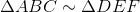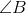is a right angle;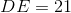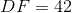.

Which is the greater quantity?

(a)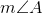(b)Possible Answers:

(a) and (b) are equal.

It is impossible to tell from the information given.

(b) is greater.

(a) is greater.

Correct answer:

(a) and (b) are equal.

Explanation:. Corresponding angles of similar triangles are congruent, so sinceis a right angle, so is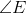The hypotenuse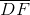of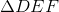is twice as long as leg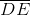; by the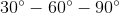Theorem,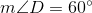. Again, by similiarity,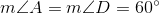.

### All ISEE Upper Level Math Resources